Volume 4, Issue 2, April 2015, Pages: 20-29

Determination of Friction Coefficient Between Straight Steel Fiber and the Concrete Fri (SSF.C)

Department of Civil and Environmental Engineering, College of Engineering, Majmaah University, Riyadh, Kingdom of Saudi Arabia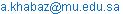Amjad Khabaz. Determination of Friction Coefficient Between Straight Steel Fiber and the Concrete Fri (SSF.C). Advances in Materials. Vol. 4, No. 2, 2015, pp. 2029. doi: 10.11648/j.am.20150402.11

Abstract: The friction forces between steel fiber and the concrete in the case of Straight Steel Fiber Reinforced Concrete (SSFRC) are considered as the main factor to generate the bonding between these two building construction materials. Three types of steel fiber are usually used in Fiber Reinforced Concrete (FRC) Straight, Dramix and Tabix. In the case of using straight steel fiber as reinforcement material to improve the resistance capacity of plain concrete under axial or flexural tension forces to obtain Steel Fiber Reinforced Concrete (SFRC), bonding forces at the interface between the steel fiber and the concrete matrix must be satisfactory. Bonding forces between these two materials are generated due to friction forces at the interface; therefore the final evaluation of the bonding forces is related with the real value of the friction forces, consequently the friction coefficient value between straight steel fiber and the concrete is important to evaluate and calculate the real value of the friction forces. This paper is devoted to introduce an experimental study about the mechanism of removing straight steel fiber from concrete matrix which is named as (pull-out test) as well as a programming simulations prepared to represent this mechanism too, these laboratory experiment and computer simulations have been used in determination process of friction coefficient value between straight steel fiber and the concrete Fri (SSF.C).

Keywords: Straight Steel Fiber Reinforced Concrete (SSFRC), Steel Fiber Reinforced Concrete (SFRC), Fiber Reinforced Concrete (FRC), Steel Fiber, Friction Coefficient Fri (SSF.C), Concrete, Pull-Out

1. Introduction

Fibers are usually used in concrete to control cracking due to plastic shrinkage and to drying shrinkage. They also reduce the permeability of concrete and thus reduce bleeding of water.

Generally different types of fibers are used in concrete such as glass fiber, carbon fiber and steel. In the case of steel fiber reinforced concrete, different types of steel fiber are usually used depending on fiber shape such as Straight, Dramix and Tabix (see Fig 1.1).

Tensile strength of steel fibers is between 400 MPa and 1500 MPa. Strength of highly carbonated steel fibers may reach about 2000 MPa. Young modulus is about 200 GPa. Fibers are isotropic or may be slightly anisotropic. Steel fiber concrete is widely used in different civil engineering applications, variety of fibers is commercially available, and this is the reason why practical engineers are more familiar with such fibers applications .

In the case of straight steel fiber in concrete only friction forces between the fiber and the concrete resist the pull-out forces, and the mechanical behaviour of steel fiber in the concrete will control the cracks. Therefore determination of friction coefficient between steel fiber and the concrete is important to evaluate the frictional part of pull-out resistance, where this frictional part represents the main part of pull-out mechanism in the case of straight steel fibers.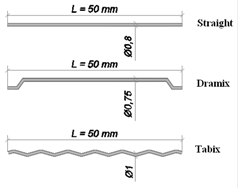Figure 1.1. Types of steel fibers .

Laboratory experiment of pull-out tests of straight steel fiber embedded into concrete matrix as real sample is prepared using oval metallic mould (see Fig 1.2).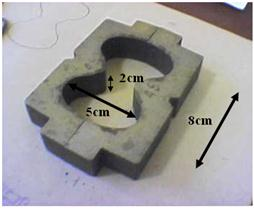Figure 1.2. Steel mould used to prepare pull-out sample .

Whereas various programming simulations as virtual samples using finite element method to represent the mechanism of removing straight steel fiber from concrete matrix under special conditions (see Fig 1.3).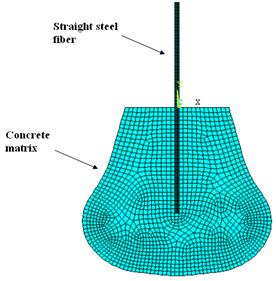Figure 1.3. Finite element model of pull-out simulation.

Results of laboratory pull-out experiment give a real description of mechanical behaviour of straight steel fiber under the influence of external tension applied load, where the sliding part of force-displacement curve will be used to determine the friction coefficient, whereas programming simulations are used to obtain several force-displacement curves using various expected values of friction coefficient between glass fiber and the concrete.

Comparison laboratory pull-out curve with several force-displacement curves from programming simulations will lead to obtain the right value of friction coefficient between straight steel fiber and the concrete.

2. Materials and Methods

2.1. Pull-Out Test Stages

Pull-out test can be divided into three stages (see Fig 2.1.1), the first stage represents the case of perfect adhesion mode where the bonding forces resist the applied tension load which is trying to extract the fiber from the concrete (see Fig 2.1.2), second stage might be named as debonding stage starts when the tension applied load reaches critical value where the bonding strength starts to diminish; then partial debonding progressively appears at the interface (see Fig 2.1.3) until full interfacial debonding situation happens; then the third pull-out stage starts where the frictional sliding mode can be shown (see Fig 2.1.4), this third stage is necessary to calculate the friction factor during the outwards-sliding motion of the fiber from the concrete matrix. Therefore we will choose an experimental single straight steel fiber pull-out curve and also prepare suitable programming simulations using different values of friction coefficient, from experiments and simulations various force-displacement curves will be obtained, then conformity between the experimental results and programming simulations will lead to fond the right value of friction coefficient between straight steel fiber and the concrete.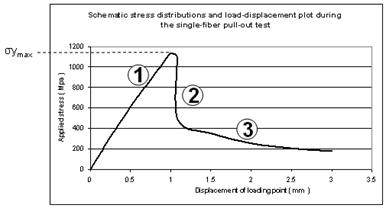Figure 2.1.1. Pull-out test three stages.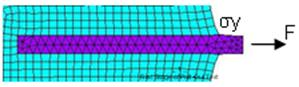Figure 2.1.2. First stage of pull-out test (perfect adhesion).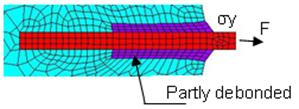Figure 2.1.3. Second stage of pull-out test (partial debonding).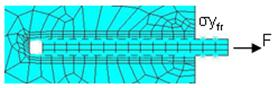Figure 2.1.4. Third stage of pull-out test (frictional sliding).

2.2. Experimental Pull-Out Test Results

An experimental sample of single straight steel fiber (Length L = 5cm, Diameter = 0.8mm) is prepared in the lab, where this single steel fiber is embedded 0.5L = 2.5cm into concrete matrix, and the concrete matrix is prepared according to the concrete mix components as shown in the table 2.2.1.

Table 2.2.1. Components of the concrete matrix used in pull-out tests.

 Designation: F50a 1 m3 1 liter Components CEM II 42.5 A-V 556 556.00 Sand 0.3/2.5 mm 863 863.00 Sand 0/0.5 mm 288 288.00 Filler (Dolomite powder) 150 150.00 Microsilica 81 81.00 Water 247.0 247.00 SIKA EVO 26 9.00 9.00 SIKA AER S (10%) 2.00 2.00 Fiber: Straight steel 0.00 0.00 Total fibers: 0.00 0.00 Fibers % 0.00 0.00 Total: 2196.00 2196.00

Stress-displacement curve was obtained according to pull-out test (see Fig 2.2.1, 2.2.2), where the forces converted to be stresses.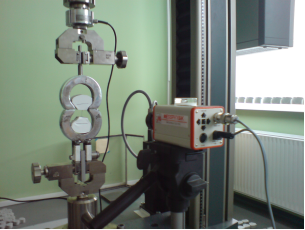Figure 2.2.1. Laboratory pull-out experiment.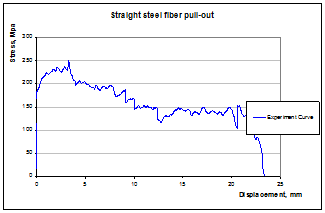Figure 2.2.2. Pull-out curve of straight steel fiber embedded 0.5L = 2.5 cm into concrete matrix.

2.3. Programming Simulations Pull-Out Test Results

Several simulations are prepared for each expected value of friction coefficient between the straight steel fiber and the concrete (0.05; 0.1; 0.15; 0.2 respectively), also for each expected value of friction coefficient different values of displacement have been applied according to the experimental displacement domain of the frictional sliding part (3.644 mm; 5.694 mm; 13.673 mm; 19.549 mm; 21.595 mm respectively) (see Fig 2.3.1, 2.3.2, 2.3.3, 2.3.4, 2.3.5). Elasticity modulus E and Poison's ratio υ of the concrete matrix and steel fiber are used in simulations as follows:

Concrete matrix E = 30 000 MPa, υ = 0.2

Steel fiber E = 200 000 MPa, υ = 0.2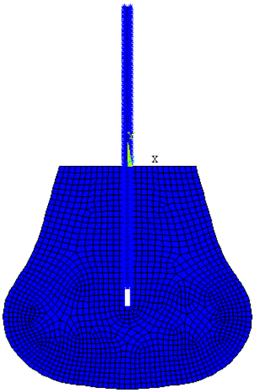Figure 2.3.1. Contact element simulation of steel fiber displacement equal 3.644mm within concrete matrix.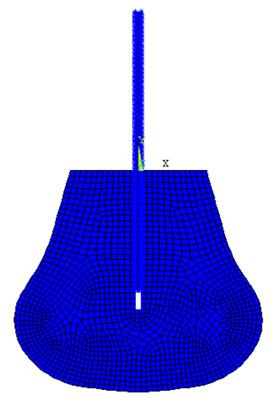Figure 2.3.2. Contact element simulation of steel fiber displacement equal 5.694mm within concrete matrix.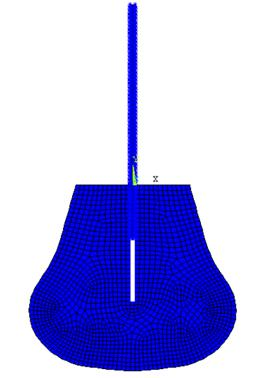Figure 2.3.3. Contact element simulation of steel fiber displacement equal 13.673mm within concrete matrix.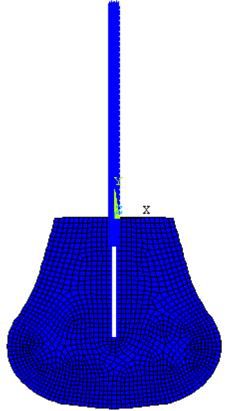Figure 2.3.4. Contact element simulation of steel fiber displacement equal 19.549mm within concrete matrix.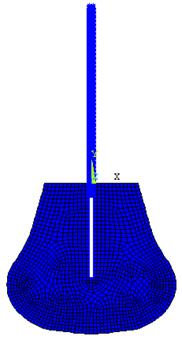Figure 2.3.5. Contact element simulation of steel fiber displacement equal 21.595mm within concrete matrix.

3. Results and Discussion

Analysis each case of previous simulations in Figures (2.3.1, 2.3.2, 2.3.3, 2.3.4, 2.3.5) separately will give contours of y-stress distribution which might be used to obtain the maximum stress in Y direction. This analysis must be applied for each case under various values of friction coefficient between the steel fiber and the concrete as expected values such as 0.05, 0.10, 0.15, and 0.20. For each case of previous simulations relevant to each expected value of friction coefficient maximum y-stress can be found, consequently for each value of friction coefficient separated curve between stresses and displacement can be drawn.

Applying this procedure on the case of Fri=0.1 as expected value of friction coefficient for each value of the limited displacement relevant to previous simulations (3.644mm, 5.694mm, 13.673mm, 19.549mm and 21.595mm) will give y-stress as the following (see Fig 3.1, 3.2, 3.3, 3.5, 3.5).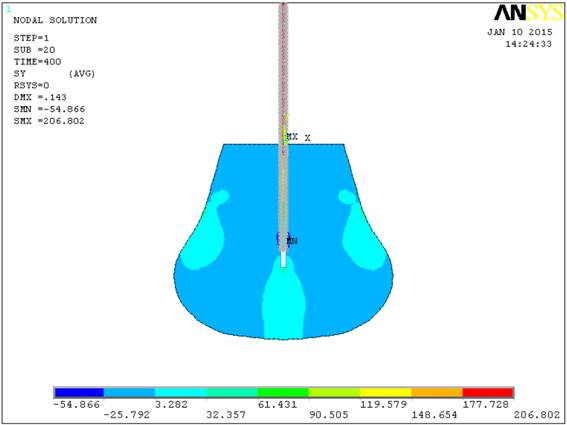Figure 3.1.a. Stress distribution of steel fiber simulation within concrete matrix (displacement = 3.644mm, Friction coefficient = 0.1.Figure 3.1.b. Stress distribution of steel fiber simulation within concrete matrix (displacement = 3.644mm, Friction coefficient = 0.1.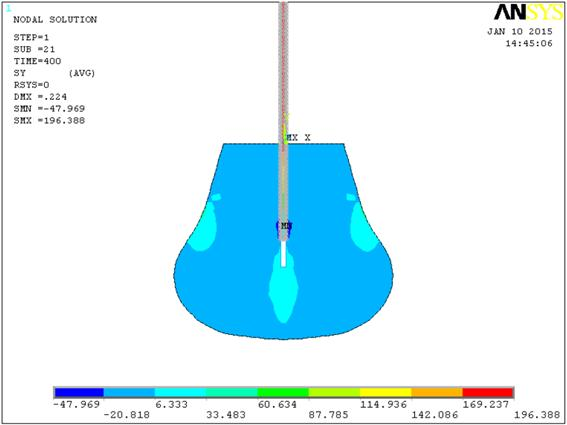Figure 3.2. Stress distribution of steel fiber simulation within concrete matrix (displacement = 5.694mm, Friction coefficient = 0.1.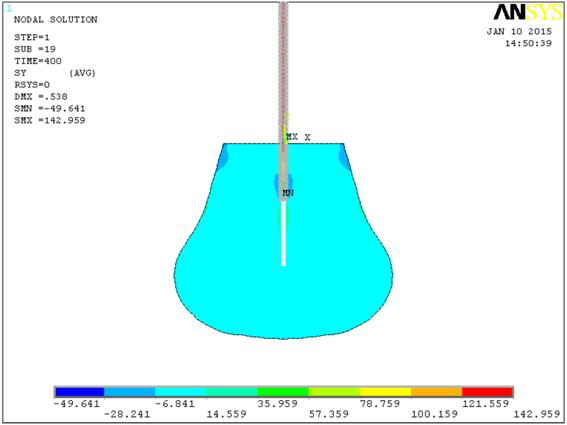Figure 3.3. Stress distribution of steel fiber simulation within concrete matrix (displacement = 13.673mm, Friction coefficient = 0.1.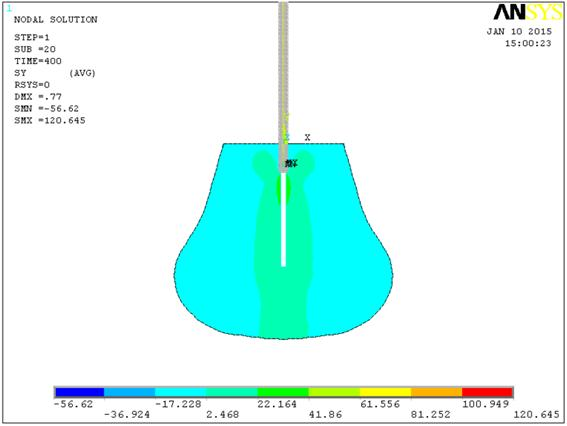Figure 3.4. Stress distribution of steel fiber simulation within concrete matrix (displacement = 19.549mm, Friction coefficient = 0.1.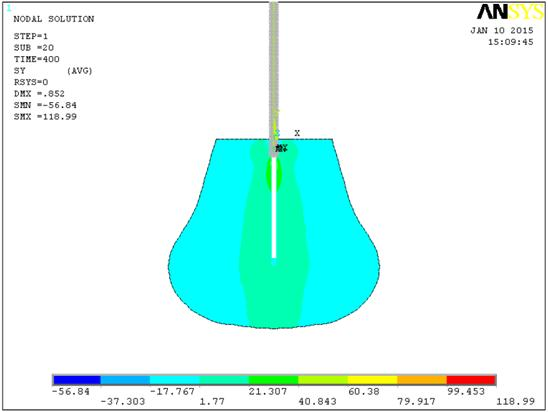Figure 3.5. Stress distribution of steel fiber simulation within concrete matrix (displacement = 21.595mm, Friction coefficient = 0.1.

Stress distribution results can be used to find the maximum y-stress of each simulation in the case of friction coefficient Fri(SSF.C) = 0.1 as it is shown in the following table (see Table 3.1).

Table 3.1. Maximum y-stress of steel fiber in the case of friction coefficient Fri(SSF.C) = 0.1and different displacement values.

 Displacement (mm) (in) 3.644 0.143 5.694 0.224 13.673 0.538 19.549 0.770 21.595 0.852 Simulation y-stress (MPa) Fri(SSF.C) = 0.10 206.802 196.388 142.959 120.645 118.990

The relation between maximum y-stresses and limited displacement according to the data in table 3.1 can be drawn as it is shown in Figure 3.5.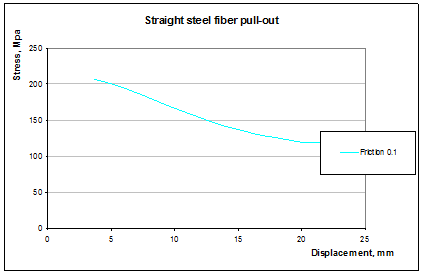Figure 3.6. Maximum y-stress of steel fiber in the case of friction coefficient Fri(SSF.C) = 0.1and different displacement values.

Repeating similar procedure for other expected values of friction coefficient such as 0.05, 0.15, and 0.20 will lead to obtain the table 3.2 and draw curves as it is shown in Fig 3.7.

Table 3.2. Maximum y-stress of steel fiber in various cases of friction coefficient values (0.05, 0.10, 0.15, 0.20).

 Displacement (mm) (in) 3.644 0.143 5.694 0.224 13.673 0.538 19.549 0.770 21.595 0.852 Experimental applied tension force  (N) 108.360 95.879 66.750 72.056 65.374 Experimental axial stress (MPa) 215.857 190.994 132.969 143.537 130.227 Simulation y-stress (MPa) Friction coefficient Fri(SSF.C) 0.20 418.835 378.922 273.46 162.109 156.361 0.15 304.723 289.112 207.697 141.019 137.579 0.10 206.802 196.388 142.959 120.645 118.990 0.05 110.752 104.215 86.600 100.840 100.820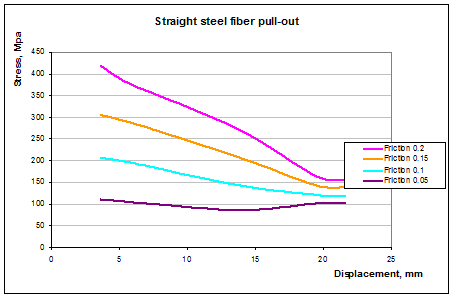Figure 3.7. Maximum y-stress of steel fiber in various cases of friction coefficient values (0.05, 0.10, 0.15, 0.20).

Comparing the experimental curve in Figure 2.2.1 with the simulation curves in Figure 3.7 leads to predicting the real value of the friction coefficient Fri(SSF.C) between straight steel fiber and the concrete to be around 0.1 (see Fig 3.8).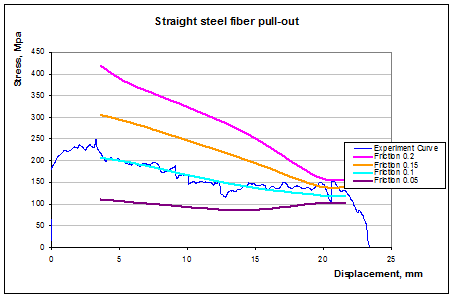Figure 3.8. Comparison between experimental results and programming simulations result.

The experimental curve is closer to the simulation curve of friction coefficient = 0.1, therefore the predicted value of friction coefficient between the straight steel fiber and the concrete is 0.1.

Friction Coefficient Fri(SSF.C) = 0.1

4. Conclusions

Ÿ Predicted value of friction coefficient between straight steel fiber and the concrete equal to 0.1.

Ÿ Pull-out test is divided into three stages according to debonding level at the interface of steel fiber and the concrete.

Ÿ Sliding part of experimental pull-out test represents the stage which must be used to find the expected value of friction coefficient.

Ÿ Programming simulations of pull-out test were very important to determine the expected ranges of friction coefficient values.

Ÿ Evaluation of bonding forces between straight steel fiber and the concrete is relevant to the value of friction coefficient between these two building construction materials.

References

1. Amjad Khabaz, "Non-Metallic Fiber Reinforced Concrete", LAP LAMBERT Academic Publishing, 2014, ISBN 978-3-659-50914-8.
2. A.Krasnikovs V.Lapsa and M.Eiduks, "NON-TRADITIONAL REINFORCEMENT FOR CONCRETE COMPOSITES - STATE OF THE ART ", Riga Technical University, Latvia, 2007.
3. Amjad Khabaz. Determination of Friction Coefficient between Glass Fiber and the Concrete Fri(GF.C). International Journal of Materials Science and Applications. Vol. 3, No. 6, 2014, pp. 321-324. doi: 10.11648/j.ijmsa.20140306.17.
4. A.Krasnikovs, A. Khabaz, G.Shahmenko and V.Lapsa. GLASS AND CARBON FIBER CONCRETE MICROMECHANICAL AND MACROMECHANICAL PROPERTIES // Sc. Proceedings of Riga Technical University, Transport and Engineering, 6 Vol. 28, Latvia, 2008, p.132-141.
5. Krasņikovs A., Kononova O., Khabaz A., Vība J. Fiberconcrete Non-Linear Fracture Control through Fresh Concrete Flow Numerical Simulation // Journal of Vibroengineering. Vol.12, Iss.2. (2010) pp 149-160.
6. Fachvereinigung Faserbeton e.V.-. Glassfibre reinforced concrete: practical design and structural analysis // Beton-Verl., 1995.
7. D. Hull and T. W. Clyne. An Introduction to Composite Materials // Second Edition, 1996, Cambridge University Press.
8. RILEM Symposium 1978, Testing and Test Methods of Fibre Cement Composites, edited by N. R. Swarmy, The Construction Press, Lancaster, 1978.
9. S. Frondistou-Yannas. Flexural strength of concrete with randomly oriented glass fibers // Magazine of concrete research: Vol. 29, No. 100: September 1977.

 Contents 1. 2. 2.1. 2.2. 2.3. 3. 4.
Article ToolsAbstractPDF(830K)